## GATE 2014 [Set-3]

 Question 1

While trying to collect(I) an envelope from under the table(II), Mr. X fell down (III) and was losing consciousness (IV) Which one of the above underlined parts of the sentence is NOT appropriate?

 A I B II C III D IV
Aptitude       Verbal
Question 1 Explanation:
Losing consiousness represents that it is a continuous process of losing the consiousness. It is not appropriate, he just lost the consiousness.
 Question 2

If she _____________ how to calibrate the instrument, she ______________ done the experiment.

 A knows, will have B knew, had C had known, could have D should have known, would have
Aptitude       Verbal
Question 2 Explanation: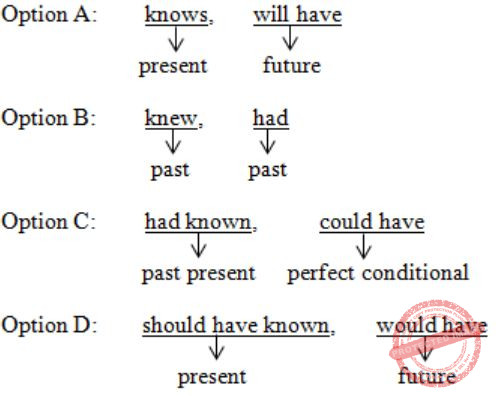Rule: If + past perfect then result to be perfect conditional (or) perfect continuous condition.
 Question 3

Choose the word that is opposite in meaning to the word “coherent”.

 A sticky B well-connected C rambling D friendly
Aptitude       Verbal
Question 3 Explanation:
Coherent = logical and consistent
Rambling = lengthy and confused (or) inconsequential
 Question 4

Which number does not belong in the series below?

`2, 5, 10, 17, 26, 37, 50, 64`
 A 17 B 37 C 64 D 26
Aptitude       Numerical
Question 4 Explanation:
2, 5, 10, 17, 26, 37, 50, 64
2 = 12+1
5 = 22+1
10 = 32+1
17 = 42+1
26 = 52+1
37 = 62+1
50 = 72+1
64 = 82+0
64 does not belong to the series.
 Question 5

The table below has question-wise data on the performance of students in an examination. The marks for each question are also listed. There is no negative or partial marking in the examination.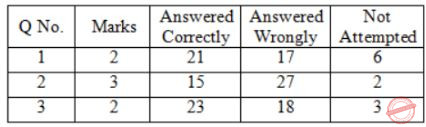What is the average of the marks obtained by the class in the examination?

 A 1.34 B 1.74 C 3.02 D 3.91
Aptitude       Numerical
Question 5 Explanation:
Number of students = 21+17+6 (or) 15+27+2 (or) 23+18+3 = 44
Total marks obtained = (21×2)+(15×3)+(23×2) = 133
Average marks = 133/44 = 3.02
 Question 6

A dance programme is scheduled for 10.00 a.m. Some students are participating in the programme and they need to come an hour earlier than the start of the event. These students should be accompanied by a parent. Other students and parents should come in time for the programme. The instruction you think that is appropriate for this is

 A Students should come at 9.00 a.m. and parents should come at 10.00 a.m. B Participating students should come at 9.00 a.m. accompanied by a parent, and other parents and students should come by 10.00 a.m. C Students who are not participating should come by 10.00 a.m. and they should not bring their parents. Participating students should come at 9.00 a.m. D Participating students should come before 9.00 a.m. Parents who accompany them should come at 9.00 a.m. All others should come at 10.00 a.m.
Aptitude       Numerical
Question 6 Explanation:
Students who are particularly in the program and they need to come an hour earlier i.e., 09.00 am because the program is start of 10.00 am.
→ All other students and parents should come in time for the programme i.e. 10.00 am.
→ Option B is correct answer.
→ In option D, they gave, all other should come at 10.00 am that includes student's parents, staff and all others. So this is not correct option.
 Question 7

By the beginning of the 20th century, several hypotheses were being proposed, suggesting a paradigm shift in our understanding of the universe. However, the clinching evidence was provided by experimental measurements of the position of a star which was directly behind our sun.

Which of the following inference(s) may be drawn from the above passage?

(i) Our understanding of the universe changes based on the positions of stars
(ii) Paradigm shifts usually occur at the beginning of centuries
(iii) Stars are important objects in the universe
(iv) Experimental evidence was important in confirming this paradigm shift
 A (i), (ii) and (iv) B (iii) only C (i) and (iv) D (iv) only
Aptitude       Verbal
Question 7 Explanation:
Paradigm shift means a fundamental change in approach to something. This change, as per the question, was verified with the experimental measurements of the position of a star that was behind the sun.
(i) is incorrect as it generalizes the experimental evidence of the star and assumes it to be always true, which may not be the case every time.
(ii) and (iii) is anyway wrong.
 Question 8

The Gross Domestic Product (GDP) in Rupees grew at 7% during 2012-2013. For international comparison, the GDP is compared in US Dollars (USD) after conversion based on the market exchange rate.  During the period 2012-2013 the exchange rate for the USD increased from Rs. 50/ USD to Rs. 60/ USD. India’s GDP in USD during the period 2012-2013

 A increased by 5% B decreased by 13% C decreased by 20% D decreased by 11%
Aptitude       Numerical
Question 8 Explanation:
Let,
GDP in rupees = x
GDP in dollars = x/50
Increase in GDP in rupees = 7%
∴ New GDP in rupees = 1.07x
New GDP in dollars = 1.07x/60
Change = ((1.07x/60) - (x/50))/(x/50) = -6.5/60 = -10.83%
As it is negative, the value has decreased.
GDP in VSD has decreased by 11%.
 Question 9

The ratio of male to female students in a college for five years is plotted in the following line graph. If the number of female students in 2011 and 2012 is equal, what is the ratio of male students in 2012 to male students in 2011?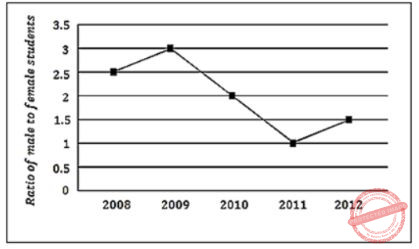A 1:1 B 2:1 C 1.5:1 D 2.5:1
Aptitude       Numerical
Question 9 Explanation:
Given,
Male to female students ratio in 2011 = 1 : 1
Male to female students ratio in 2012 = 1.5 : 1 = 3 : 2⇒ M1/F1 = 1:1
M1 = F1 ------- (1)
⇒ M2/F2 = 1:1
2M2 = 3F2 ------- (2)
Given,
F1 = F2 ------- (3) From (1) & (2)
M1/M2 = F1/(3F2/2) = 2F1/3F2
But from (3)
M1/M2 = 2/3
We need to find
M2 : M1 = 3 : 2 = 1.5 : 1
 Question 10

Consider the equation: (7526)8 - (Y) = (4364)8, where (X)N stands for X to the base N. Find Y.

 A 1634 B 1737 C 3142 D 3162
Aptitude       Numerical
Question 10 Explanation:
(7526)8 - (Y)8 = (4364)8
⇒ 1/8 = (7526)8 - (4364)8
Base 8 ⇒ 0 to 7 digitsWhen you are borrowing you will add the value of the base, hence 2 becomes (2+8) = 10
Y = 3142
 Question 11

Consider the following statements:

```P: Good mobile phones are not cheap
Q: Cheap mobile phones are not good
L: P implies Q
M: Q implies P
N: P is equivalent to Q
```

Which one of the following about L, M, and N is CORRECT?

 A Only L is TRUE. B Only M is TRUE. C Only N is TRUE. D L, M and N are TRUE.
Engineering-Mathematics       Prepositional-Logic
Question 11 Explanation:
In the given statements observe that "not cheap" & cheap, "good & not good" are used.
So, given statement can be sub divided such that we can utilize the negation of this atomic statements.
Suppose, X is Good mobile and Y is cheap then
P: (Good(x) → ~cheap(x)) → (~good(x) ∨ ~cheap(x))
Q: cheap(x) → ¬good(x) ⟺ ((¬cheap(x) ∨ good(x)) ⟺ ¬good(x) ∨ ¬cheap(x))
All these are contra positive.
All L, M, N are true.
 Question 12

Let X and Y be finite sets and f: X→Y be a function. Which one of the following statements is TRUE?

 A For any subsets A and B of X, |f(A ∪ B)| = |f(A)|+|f(B)| B For any subsets A and B of X, f(A ∩ B) = f(A) ∩ f(B) C For any subsets A and B of X, |f(A ∩ B)| = min{ |f(A)|,f|(B)|} D For any subsets S and T of Y, f -1 (S ∩ T) = f -1 (S) ∩ f -1 (T)
Engineering-Mathematics       Set-Theory
Question 12 Explanation:
The function f: x→y.
We need to consider subsets of 'x', which are A & B (A, B can have common elements are exclusive).
Similarly S, T are subsets of 'y'.To be a function, each element should be mapped with only one element.
(a) |f(A∪B)| = |f(A)|+|f(B)|
|{a,b,c}|∪|{c,d,e}| = |{a,b,c}| + |{c,d,e}|
|{a,b,c,d,e}| = 3+3
5 = 6 FALSE
(d) To get inverse, the function should be one-one & onto.
The above diagram fulfills it. So we can proceed with inverse.
f-1 (S∩T ) = f-1 (S)∩f-1 (T)
f-1 (c) = f-1 ({a,b,c})∩f-1 ({c,d,e})
2 = {1,2,3}∩{2,4,5}
2 = 2 TRUE
 Question 13

Let G be a group with 15 elements. Let L be a subgroup of G. It is known that L ≠ G and that the size of L is at least 4. The size of L  is __________.

 A 5 B 6 C 7 D 8
Engineering-Mathematics       Set-Theory
Question 13 Explanation:
Lagrange's theorem, in the mathematics of group theory, states that for any finite group G, the order (number of elements) of every subgroup H of G divides the order of G.
So, 15 is divided by {1, 3, 5, 15}.
As minimum is 4 and total is 15, we eliminate 1,3,15.
 Question 14

Which one of the following statements is TRUE about every n × n matrix with only real eigenvalues?

 A If the trace of the matrix is positive and the determinant of the matrix is negative, at least one of its eigenvalues is negative. B If the trace of the matrix is positive, all its eigenvalues are positive. C If the determinant of the matrix is positive, all its eigenvalues are positive. D If the product of the trace and determinant of the matrix is positive, all its eigenvalues are positive.
Engineering-Mathematics       Linear-Algebra
Question 14 Explanation:
The sum of the n eigenvalues of A is the same as the trace of A (that is, the sum of the diagonal elements of A).
• The product of the n eigenvalues of A is the same as the determinant of A. •
A: Yes, for sum to be negative there should be atleast one negative number.
B: There can be one small negative number and remaining positive, where sum is positive.
C: Product of two negative numbers is positive. So, there no need of all positive eigen values.
D: There is no need for all eigen values to be positive, as product of two negative numbers is positive.
 Question 15

If V1 and V2 are 4-dimensional subspace of a 6-dimensional vector space V, then the smallest possible dimension of V1∩V2   is ______.

 A 2 B 3 C 4 D 5
Engineering-Mathematics       Set-Theory
Question 15 Explanation:
In a 6 dimensional vector space, sub space of 4 dimensional subspace V1, V2 are provided. Then the V1∩V2?
For eg: a two dimensional vector space have x, y axis. For dimensional vector space, it have x, y, z axis.
In the same manner, 6 dimensional vector space has x, y, z, p, q, r (assume).
Any subspace of it, with 4 dimensional subspace consists any 4 of the above. Then their intersection will be atmost 2.
[{x,y,z,p} ∩ {r,q,p,z}] = #2
V1 ∩ V2 = V1 + V2 - V1 ∪ V2 = 4 + 4 + (-6) = 2
 Question 16

If, then the value of k is equal to ________.

 A 4 B 5 C 6 D 7
Engineering-Mathematics       Calculus
Question 16 Explanation:
The graph x.Sinx from 0 to 2π isWe have |xSinx|,We can observe that it is positive from 0 to π and negative in π to 2π.
To get positive value from π to 2π we put ‘-‘ sign in the (π, 2π)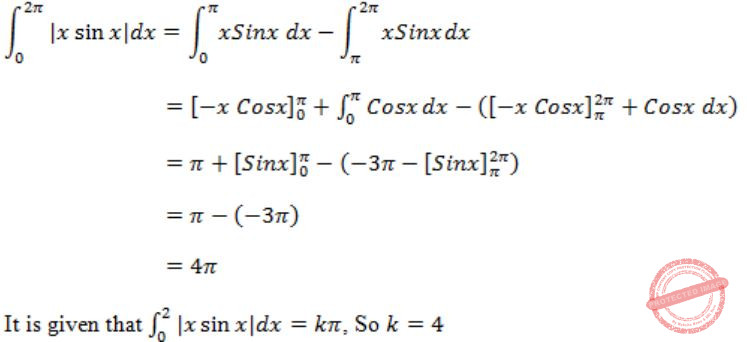Question 17

Consider the following minterm expression for F:

`  F(P,Q,R,S) = Σ0,2,5,7,8,10,13,15  `

The minterms 2, 7, 8 and 13 are 'do not care' terms. The minimal sum-of-products form for F is:

 A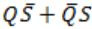BC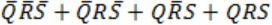DDigital-Logic-Design       Number-Systems
Question 17 Explanation:Question 18

Consider the following combinational function block involving four Boolean variables x, y, a, b where x, a, b are inputs and y is the output.

```f (x, y, a, b)
{
if (x is 1) y = a;
else y = b;
}
```

Which one of the following digital logic blocks is the most suitable for implementing this function?

 A Full adder B Priority encoder C Multiplexor D Flip-flop
Digital-Logic-Design       Boolean-Variables
Question 18 Explanation:
A 2x1 Multiplexer is most suitable for implementing the function.
x is the select line, I0 is 'b' and I1 is a.
The output line, y = xa + x’b
 Question 19

Consider the following processors (ns stands for nanoseconds). Assume that the pipeline registers have zero latency.

P1: Four-stage pipeline with stage latencies 1 ns, 2 ns, 2 ns, 1 ns.
P2: Four-stage pipeline with stage latencies 1 ns, 1.5 ns, 1.5 ns, 1.5 ns.
P3: Five-stage pipeline with stage latencies 0.5 ns, 1 ns, 1 ns, 0.6 ns, 1 ns.
P4: Five-stage pipeline with stage latencies 0.5 ns, 0.5 ns, 1 ns, 1 ns, 1.1 ns.

Which processor has the highest peak clock frequency?

 A P1 B P2 C P3 D P4
Question 19 Explanation:
Clock period (CP) = max stage delay + overhead
So CPP1 = Max(1,2,2,1) = 2ns
CPP2 = Max(1,1.5,1.5,1.5) = 1.5ns
CPP3 = Max(0.5,1,1,0.6,1) = 1ns
CPP4 = Max(0.5,0.5,1,1,1.1)=1.1ns
As frequency ∝ 1/C.P, so least clock period will give the highest peak clock frequency.
So, fP3 = 1/1ns = 1GHz
 Question 20

Let A be a square matrix of size n x n. Consider the following program. What is the expected output?

```C = 100
for i = 1 to n do
for j = 1 to n do
{
Temp = A[i][j] + C
A[i][j] = A[j][i]
A[j][i] = Temp - C
}
for i = 1 to n do
for j = 1 to n do
Output(A[i][j]);
```
 A The matrix A itself B Transpose of the matrix A C Adding 100 to the upper diagonal elements and subtracting 100 from lower diagonal elements of A D None of the above
Programming       Programming
Question 20 Explanation:
Letbe a small matrix.
For first row iteration, it get swapped and becomesFor second row iteration, it comes to the original position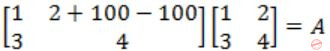=A
So, it is the same matrix A.
 Question 21

The minimum number of arithmetic operations required to evaluate the polynomial P(X) = X5 + 4X3 + 6X + 5 for a given value of X, using only one temporary variable is ________.

 A 7 B 8 C 9 D 10
Programming       Arithmetic-Expressions
Question 21 Explanation:
The minimum number of arithmetic operations required to evaluate
P(X) = x5+4x3+6x+5
= x(x4+4x2+6)+5
= x(x(x3+4x)+6)+5
= x(x(x(x2+4))+6)+5
= x(x(x(x(x)+4))+6)+5
4 + 3 = 7
 Question 22

Consider the following rooted tree with the vertex labeled P as t he root:The order in which the nodes are visited during in-order traversal is

 A SQPTRWUV B SQPTUWRV C SQPTWUVR D SQPTRUWV
Algorithms       Tree Traversal
Question 22 Explanation:
The tree can be redrawn asInorder Traversal: Left, Root, Middle, Right.
So, during inorder traversal whenever we visit the node second time then print it.
So, output will be,
S Q P T R W U V
 Question 23

Suppose depth first search is executed on the graph below starting at some unknown vertex. Assume that a recursive call to visit a vertex is made only after first checking that the vertex has not been visited earlier. Then the maximum possible recursion depth (including the initial call) is _________.A 19 B 20 C 21 D 22
Algorithms       Graphs
Question 23 Explanation:Note: We should not consider backtrack edges, it reduces recursion depth in stack.
So the maximum possible recursive depth will be 19.Question 24

You have an array of n elements. Suppose you implement quicksort by always choosing the central element of the array as the pivot. Then the tightest upper bound for the worst case performance is

 A O(n2) B O(n log n) C Θ(n log⁡n) D O(n3)
Algorithms       Sorting
Question 24 Explanation:
The Worst case time complexity of quick sort is O (n2). This will happen when the elements of the input array are already in order (ascending or descending), irrespective of position of pivot element in array.
 Question 25

The length of the shortest string NOT in the language (over Σ = {a b,} of the following regular is expression is ______________.

`     a*b*(ba)*a* `
 A 3 B 4 C 5 D 6
Theory-of-Computation       Regular Languages and Finite Automata
Question 25 Explanation:
The regular expression generate all the strings of length 0 , 1 and 2
{ϵ, a, b, aa, ab, ba, bb}
Let’s check all the string of length 3.
The given regular expression generates {aaa, aab, aba, abb, baa, bba, bbb}
But it doesn’t generate the string “bab”, hence the shortest string not generated by regular expression has length 3 (string “bab”).
 Question 26

Let Σ be a finite non-empty alphabet and let 2Σ* be the power set of Σ*. Which one of the following is TRUE?

 A Both 2Σ* and Σ* are countable B 2Σ* is countable and Σ* is uncountable C 2Σ* is uncountable and Σ* is countable D Both 2Σ* and Σ* are uncountable
Theory-of-Computation       Decidability-and-Undecidability
Question 26 Explanation:
If = {0,1} then Σ* = {ϵ, 0, 1, 00, 01, 10, 11, 000, ...............}
Since we can enumerate all the strings of Σ*, hence Σ* is countable (countable infinite).
While 2Σ* is uncountable, it has been already proved by using Diagonalization method.
 Question 27

One of the purposes of using intermediate code in compilers is to

 A make parsing and semantic analysis simpler. B improve error recovery and error reporting. C increase the chances of reusing the machine-independent code optimizer in other compilers. D improve the register allocation.
Compiler-Design       Code-Generation-and-Code-Optimization
Question 27 Explanation:
Intermediate code is generated after semantic analysis. The intermediate code is independent of machine. By converting source code to intermediate code a machine independent code optimizer may be written. Thus increase the chances of reusing the machine-independent code optimizer in other compilers.
 Question 28

Which of the following statements are CORRECT?

1) Static allocation of all data areas by a compiler makes it impossible to implement recursion.
2) Automatic garbage collection is essential to implement recursion.
3) Dynamic allocation of activation records is essential to implement recursion.
4) Both heap and stack are essential to implement recursion.
 A 1 and 2 only B 2 and 3 only C 3 and 4 only D 1 and 3 only
Compiler-Design       General
Question 28 Explanation:
The statement, static allocation of all data areas by a compiler makes it impossible to implement recursion is true, as recursion requires memory allocation at run time, so it requires dynamic allocation of memory. Hence, Dynamic allocation of activation records is essential to implement recursion is also a true statement.
 Question 29

In the context of modular software design, which one of the following combinations is desirable?

 A High cohesion and high coupling B High cohesion and low coupling C Low cohesion and high coupling D Low cohesion and low coupling
Software-Engineering       SE
Question 29 Explanation:
Note: Out of syllabus
Cohesion is a measure of internal strength within a module, whereas coupling is a measure of inter dependency among the modules. So in the context of modular software design there should be high cohesion and low coupling.
 Question 30

A system uses 3 page frames for storing process pages in main memory. It uses the Least Recently Used (LRU) page replacement policy. Assume that all the page frames are initially empty. What is the total number of page faults that will occur while processing the page reference string given below?

`4, 7, 6, 1, 7, 6, 1, 2, 7, 2`
 A 6 B 7 C 8 D 9
Compiler-Design       Page-Replacement-Algorithm
Question 30 Explanation:
6 page faults will occur using LRU.
 Question 31

What is the optimized version of the relation algebra expression πA1A2F1F2(r)))), where A1, A2 are sets of attributes in  with A1 ⊂ A2 and F1, F2 are Boolean expressions based on the attributes in r?

 A πA1 (σ(F1∧F2) (r)) B πA1 (σ(F1∨F2) (r)) C πA2 (σ(F1∧F2) (r)) D πA2 (σ(F1∨F2) (r))
Database-Management-System       ER-Model
Question 31 Explanation:
 Since A1 ⊂ A2 will get only attribute A1 as it is in the outside. So we can remove project A2.
 Two Selects with Boolean expression can be combined into one select with AND of two Boolean expressions.
 Question 32

A prime attribute of a relation scheme is an attribute that appears

 A in all candidate keys of R. B in some candidate key of R. C in a foreign key of R. D only in the primary key of R .
Database-Management-System       ER-Model
Question 32 Explanation:
A prime attribute of a relation scheme R is an attribute that appears in some candidate keys of R. Need not to appear in all the candidate keys.
Ex: AB, BC, CD are candidate keys of R(ABCD). In the FDs set one attribute may not be part of all the FDs.
 Question 33

In the following pairs of OSI protocol layer/sub-layer and its functionality, the INCORRECT pair is

 A Network layer and Routing B Data Link Layer and Bit synchronization C Transport layer and End-to-end process communication D Medium Access Control sub-layer and Channel sharing
Computer-Networks       OSI-Layers
Question 33 Explanation:
(a) One of the main functionality of Network Layer is Routing. So Option (a) is CORRECT.
(b) Bit Synchronization is always handled by Physical Layer of OSI model but not Data Link Layer. So Option (b) is INCORRECT.
(c) End – to – End Process Communication is handled by Transport Layer. So Option (c) is CORRECT.
(d) MAC sub layer have 3 types of protocols (Random, Controlled and Channelized Access).
 Question 34

A bit-stuffing based framing protocol uses an 8-bit delimiter pattern of 01111110. If the output bit-string after stuffing is 01111100101, then the input bit-string is

 A 0111110100 B 0111110101 C 0111111101 D 0111111111
Question 34 Explanation:
Given 8-bit delimiter pattern of 01111110.
Output Bit string after stuffing is 01111100101.
 Question 35

Host A (on TCP/IP v4 network A) sends an IP datagram D to host B (also on TCP/IP v4 network B). Assume that no error occurred during the transmission of D. When D reaches B, which of the following IP header field(s) may be different from that of the original datagram D?

```(i) TTL
(ii) Checksum
(iii) Fragment Offset ```
 A (i) only B (i) and (ii) only C (i) and (ii) only D (i), (ii) and (iii)
Computer-Networks       TCP
Question 35 Explanation:
While an IP Datagram is transferring from one host to another host, TTL, Checksum and Fragmentation Offset will be changed.
 Question 36

An IP router with a Maximum Transmission Unit (MTU) of 1500 bytes has received an IP packet of size 4404 bytes with an IP header of length 20 bytes. The values of the relevant fields in the header of the third IP fragment generated by the router for this packet are

```     Prefix            Output Interface
Identifier
131.16.0.0/ 12              3
131.28.0.0/ 14              5
131.19.0.0/ 16              2
131.22.0.0/ 15              1
```

The identifier of the output interface on which this packet will be forwarded is ______.

 A 1 B 2 C 3 D 4
Computer-Networks       Network-Layer
Question 36 Explanation:
Lets take, 131.22.0.0/15 Its Net Mask is 255.254.0.0.
If we do AND operation between 255.254.0.0 and given IP 131.23.151.76, gives 131.22.0.0 which is matching with interface 1.
 Question 37

Every host in an IPv4 network has a 1-second resolution real-time clock with battery backup. Each host needs to generate up to 1000 unique identifiers per second. Assume that each host has a globally unique IPv4 address. Design a 50-bit globally unique ID for this purpose. After what period (in seconds) will the identifiers generated by a host wrap around?

 A 256 B 257 C 258 D 259
Computer-Networks       IPv4-Protocol
Question 37 Explanation:
Given that each host has a globally unique IPv4 Address and we have to design 50 bit unique Id. So, 50 bit in the sense (32 + 18). So, It is clearly showing that IP Address (32 bit) followed by 18 bits.
1000 unique Ids ⇒ 1Sec
218 unique Ids ⇒ 218/1000 = 28 = 256
 Question 38

An IP router with a Maximum Transmission Unit (MTU) of 1500 bytes has received an IP packet of size 4404 bytes with an IP header of length 20 bytes. The values of the relevant fields in the header of the third IP fragment generated by the router for this packet are

 A MF bit: 0, Datagram Length: 1444; Offset: 370 B MF bit: 1, Datagram Length: 1424; Offset: 185 C MF bit: 1, Datagram Length: 1500; Offset: 370 D MF bit: 0, Datagram Length: 1424; Offset: 2960
Computer-Networks       IP-Routing-MTU
Question 38 Explanation:
Number of packet fragments = ⌈ (total size of packet)/(MTU) ⌉
So Datagram with data 4404 byte fragmented into 3 fragments.Question 39

Consider the transactions T1, T2, and T3 and the schedules S1 and S2 given below.

```T1: r1(X); r1(Z); w1(X); w1(Z)
T2: r2(Y); r2(Z); w2(Z)
T3: r3(Y); r3(X); w3(Y)
S1: r1(X); r3(Y); r3(X); r2(Y); r2(Z);
w3(Y); w2(Z); r1(Z); w1(X); w1(Z)
S2: r1(X); r3(Y); r2(Y); r3(X); r1(Z);
r2(Z); w3(Y); w1(X); w2(Z); w1(Z)
```

Which one of the following statements about the schedules is TRUE?

 A Only S1 is conflict-serializable. B Only S2 is conflict-serializable. C Both S1 and S2 are conflict-serializable. D Neither S1 nor S2 is conflict-serializable.
Computer-Networks       Transactions and concurrency control
Question 39 Explanation:
S1:No cycle, so schedule S1 is conflict serializable.
S2:There is a cycle, so S2 is not conflict serializable.
 Question 40

Consider the relational schema given below, where eId of the relation dependent is a foreign key referring to empId of the relation employee. Assume that every employee has at least one associated dependent in the dependent relation.

```employee (empId, empName, empAge)
dependent(depId, eId, depName, depAge)```

Consider the following relational algebra query:

`∏empId(employee)-∏empId(employee⋈(empId = eID)∧(empAge ≤ depAge)dependent)`

The above query evaluates to the set of empIds of employees whose age is greater than that of

 A some dependent. B all dependents. C some of his/her dependents. D all of his/her dependents.
Database-Management-System       ER-Model
Question 40 Explanation:
The inner query selects the employees whose age is less than or equal to at least one of his dependents. So, subtracting from the set of employees, gives employees whose age is greater than all of his dependents.
 Question 41

A system contains three programs and each requires three tape units for its operation. The minimum number of tape units which the system must have such that deadlocks never arise is _________.

 A 7 B 8 C 9 D 10
Question 41 Explanation:
(3*2 tape units) + 1 tape unit = 7
 Question 42

An operating system uses shortest remaining time first scheduling algorithm for pre-emptive scheduling of processes. Consider the following set of processes with their arrival times and CPU burst times (in milliseconds):The average waiting time (in milliseconds) of the processes is

 A 5.5 B 5.6 C 5.7 D 5.8
Operating-Systems       CPU-Scheduling
Question 42 Explanation: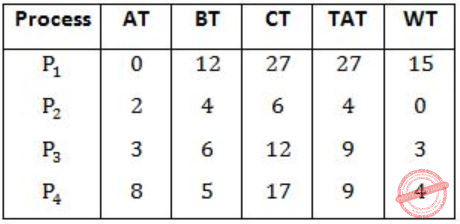WT - Waiting Time
CT - Completion Time
TAT - Turn Around Time
TAT = CT - AT < br> WT = TAT - BT
Gantt chart using Shortest remaining time first,Avg. WT = 15+0+3+4/4 = 22/4 = 5.5
 Question 43

Consider a paging hardware with a TLB. Assume that the entire page table and all the pages are in the physical memory. It takes 10 milliseconds to search the TLB and 80 milliseconds to access the physical memory. If the TLB hit ratio is 0.6, the effective memory access time (in milliseconds) is  _________.

 A 122 B 123 C 124 D 125
Operating-Systems       Memory-Management
Question 43 Explanation:
Tavg = TLB access time + miss ratio of TLB × memory access time + memory access time
= 10 + 0.4 × 80 + 80
= 10 + 32 + 80
= 122 ms
 Question 44

Consider the basic block given below.

```a = b + c
c = a + d
d = b + c
e = d - b
a = e + b```

The minimum number of nodes and edges present in the DAG representation of the above basic block respectively are

 A 6 and 6 B 8 and 10 C 9 and 12 D 4 and 4
Operating-Systems       DAG
Question 44 Explanation:
a = b + c
c = a + d
d = b + c
e = d - b = b + c - b = c
a = d - b + b = d
So, DAG representation of above basic block respectively,So, number of nodes = 6 &
number of edges = 6
 Question 45

Which one of the following problems is undecidable?

 A Deciding if a given context-free grammar is ambiguous. B Deciding if a given string is generated by a given context-free grammar. C Deciding if the language generated by a given context-free grammar is empty. D Deciding if the language generated by a given context-free grammar is finite.
Theory-of-Computation       Unecidability
Question 45 Explanation:
The problem, whether a given CFG is ambiguous is undecidable, as we don’t have any algorithm which decides it.
We have a membership algorithm which decides that whether a given string is generated by a given context-free grammar. Similarly, the problems, whether the language generated by a given context-free grammar is empty and the language generated by a given context-free grammar is finite are decidable.
 Question 46

Consider the following languages over the alphabet Σ = {0,1,c}:

L1 = {0n1n | n≥0}
L2 = {wcwr | w∈{0,1}*}
L3 = {wwr | w∈{0,1}*}

Here, wr is the reverse of the string . Which of these languages are deterministic Context-free languages?

 A None of the languages B Only L1 C Only L1 and L2 D All the three languages
Theory-of-Computation       Context-Free-and-pushdown-Automata
Question 46 Explanation:
L1 and L2 are DCFL, as we can design DPDA for them. For L1, DPDA will first push all zero’s in stack and when one appears in string, it will pop zero for every one and at the end if input string as well as stack is empty then accept the string else reject the string. Similarly for L2, DPDA will push all the string till it encounter the terminal “c” and once “c” appears in string, DPDA will ignore this “c” and then for every terminal in string (after “c”) it will pop one symbol from stack and match, if matched then pop next and continue. If didn’t match at any stage then reject the string. Since push and pop is clearly defined (i.e., every transition is deterministic), so both L1 and L2 is DCFL.
But in L3, we cannot make DPDA for it, as we cannot locate the middle of string, so DPDA for L3 is not possible. It can be accepted by NPDA only, so L3 is CFL but not DCFL.
 Question 47

Suppose you want to move from 0 to 100 on the number line. In each step, you either move right by a unit distance or you take a shortcut. A shortcut is simply a pre-specified pair of integers i, j with i < j.  Given a shortcut i, j if you are at position i on the number line, you may directly move to j. Suppose T(k) denotes the smallest number of steps needed to move from k to 100. Suppose further that there is at most 1 shortcut involving any number, and in particular from 9 there is a shortcut to 15. Let y and z be such that T(9) = 1 + min(T(y),T(z)).  Then the value of the product yz is _____.

 A 150 B 151 C 152 D 153
Theory-of-Computation       Time-Complexity
Question 47 Explanation:
T(k) is the smallest no. of steps needed to move from k to 100.
Now, it is given that
T(9) = 1 + min(T(y),T(z))
where,
T(y) = steps from y to 100
T(z) = steps from z to 100
where y and z are two possible values that can be reached from 9.
One number that can be reached from 9 is 10. Another no. is 15, the shortcut path from 9, as given in the question.
∴ The value of 'y' and 'z' are 10 and 15.
So, y × z = 10 × 15 = 150
 Question 48

Consider the decision problem 2CNFSAT defined as follows:

{Φ|Φ is a satisfiable propositional formula in CNF with atmost two literals per clause}
For example, is a Boolean formula and it is in 2CNFSAT.

The decision problem 2CNFSAT is

 A NP-Complete. B solvable in polynomial time by reduction to directed graph reachability. C solvable in constant time since any input instance is satisfiable. D NP-hard, but not NP-complete.
Theory-of-Computation       NP-Complete
Question 48 Explanation:
Note: Out of Syllabus.
 Question 49

Suppose we have a balanced binary search tree T holding n numbers.  We are given two numbers L and H and wish to sum up all the numbers in T that lie between L and H. Suppose there are m such numbers in T. If the tightest upper bound on the time to compute the sum is O(nalogbn + mclogdn), the value of a + 10b + 100c + 1000d is ____.

 A 110 B 111 C 112 D 113
Algorithms       Binary-Search-Tree
Question 49 Explanation:
It takes (log n) time to determine numbers n1 and n2 in balanced binary search tree T such that
1. n1 is the smallest number greater than or equal to L and there is no predecessor n1' of >n1 such that n1' is equal to n1.
2. n22' of n2 such that is equal to n2.
Since there are m elements between n1 and n2, it takes ‘m’ time to add all elements between n1 and n2.
So time complexity is O(log n+m)
So the given expression becomes O(n0log'n+m'log0n)
And a+10b+100c+1000d = 0+10*1+100*1+1000*1 = 10+100 = 110
Because a=0, b=1, c=1 and d=0
 Question 50

Consider a hash table with 100 slots. Collisions are resolved using chaining. Assuming simple uniform hashing, what is the probability that the first 3 slots are unfilled after the first 3 insertions?

 A (97×97×97)/1003 B (99×98×97)/1003 C (97×96×95)/1003 D (97×96×95)/(3!×1003)
Algorithms       Hashing
Question 50 Explanation:
Given Hash table consists of 100 slots.
They are picked with equal probability of Hash function since it is uniform hashing.
So to avoid the first 3 slots to be unfilled
= 97/100*97/100*97/100 =((97*97*97))⁄1003
 Question 51

Consider the pseudocode given below. The function DoSomething() takes as argument a pointer to the root of an arbitrary tree represented by the leftMostChild-rightSibling representation. Each node of the tree is of type treeNode.

```typedef struct treeNode* treeptr;
struct treeNode
{
treeptr leftMostChild, rightSibling;
};
int DoSomething (treeptr tree)
{
int value=0;
if (tree != NULL)
{
if (tree->leftMostChild == NULL)
value = 1;
else
value = DoSomething(tree->leftMostChild);
value = value + DoSomething(tree->rightSibling);
}
return(value);
}
```

When the pointer to the root of a tree is passed as the argument to DoSomething, the value returned by the function corresponds to the

 A number of internal nodes in the tree. B height of the tree. C number of nodes without a right sibling in the tree. D number of leaf nodes in the tree.
Algorithms       ree Traversals
Question 51 Explanation:
The key to solving such questions is to understand or detect where/by what condition the value (or the counter) is getting incremented each time.
Here, that condition is if (tree → leftMostchild = = Null)
⇒ Which means if there is no left most child of the tree (or the sub-tree or the current node as called in recursion)
⇒ Which means there is no child to that particular node (since if there is no left most child, there is no child at all).
⇒ Which means the node under consideration is a leaf node.
⇒ The function recursively counts, and adds to value, whenever a leaf node is encountered.
⇒ The function returns the number of leaf nodes in the tree.
 Question 52

Consider the C function given below. Assume that the array listA contains n (> 0) elements, sorted in ascending order.

```int ProcessArray(int *listA, int x, int n)
{
int i, j, k;
i = 0;
j = n-1;
do
{
k = (i+j)/2;
if (x <= listA[k])
j = k-1;
if (listA[k] <= x)
i = k+1;
}
while (i <= j);
if (listA[k] == x)
return(k);
else
return -1;
}```

Which one of the following statements about the function ProcessArray is CORRECT?

 A It will run into an infinite loop when x is not in listA. B It is an implementation of binary search. C It will always find the maximum element in listA. D It will return −1 even when x is present in listA.
Algorithms       Searching
Question 52 Explanation:
From the above code, we can identify
k = (i+j)/2;
where k keeps track of current middle element & i, j keeps track of left & right children of current subarray.
So it is an implementation of Binary search.
 Question 53

An instruction pipeline has five stages, namely, instruction fetch (IF), instruction decode and register fetch (ID/RF), instruction execution (EX), memory access (MEM), and register writeback (WB) with stage latencies 1 ns, 2.2 ns, 2 ns, 1 ns, and 0.75 ns, respectively (ns stands for nanoseconds). To gain in terms of frequency, the designers have decided to split the ID/RF stage into three stages (ID, RF1, RF2) each of latency 2.2/3 ns. Also, the EX stage is split into two stages (EX1, EX2) each of latency 1 ns. The new design has a total of eight pipeline stages. A program has 20% branch instructions which execute in the EX stage and produce the next instruction pointer at the end of the EX stage in the old design and at the end of the EX2 stage in the new design. The IF stage stalls after fetching a branch instruction until the next instruction pointer is computed. All instructions other than the branch instruction have an average CPI of one in both the designs. The execution times of this program on the old and the new design are P and Q nanoseconds, respectively. The value of P/Q is __________.

 A 1.54 B 1.55 C 1.56 D 1.57
Question 53 Explanation:
There are five stages:
Instruction Fetch (IF),
instruction decode and register fetch (ID/RF),
Instruction execution (EX),
Memory access (MEM),
and register writeback (WB)
P old design:
With stage latencies 1 ns, 2.2 ns, 2 ns, 1 ns, and 0.75 ns
Cycle time = MAX(1 ns, 2.2 ns, 2 ns, 1 ns, and 0.75 ns) = 2.2nsec
Branch penalty = 3-1 = 2 because the next instruction pointer at the end of the EX stage(which is 3rd stage) in the old design
AVG instruction execution time is
P = Tavg = (1+no. of stalls*branch penalty)*cycle time
= (1+0.20*2)2.2
P = 3.08 nsec
Q new design:
ID/RF stage is split into three stages (ID, RF1, RF2) each of latency (2.2)/3 ns = 0.73ns.
The EX stage is split into two stages (EX1, EX2) each of latency 1 ns.
The new design has a total of eight pipeline stages.
Time of stages in new design = {1ns, 0.73ns, 0.73ns, 0.73ns , 1ns, 1ns, 1ns, and 0.75ns}
Cycle time = MAX(1ns, 0.73ns, 0.73ns, 0.73ns , 1ns, 1ns, 1ns, and 0.75ns) = 1nsec
Branch penalty = 6-1 = 5 because the next instruction pointer at the end of the EX2 stage(which is 6th stage) in the new design.
AVG instruction execution time is
Q = Tavg = (1+no. of stalls*branch penality)*cycle time
= (1+0.20*5)1
Q = 2 nsec
Therefore, P/Q = 3.08/2 = 1.54
 Question 54

The memory access time is 1 nanosecond for a read operation with a hit in cache, 5 nanoseconds for a read operation with a miss in cache, 2 nanoseconds for a write operation with a hit in cache and 10 nanoseconds for a write operation with a miss in cache. Execution of a sequence of instructions involves 100 instruction fetch operations, 60 memory operand read operations and 40 memory operand write operations. The cache hit-ratio is 0.9.  The average memory access time (in nanoseconds) in executing the sequence of instructions is   __________.

 A 1.68 B 1.69 C 1.7 D 1.71
Operating-Systems       Memory-Management
Question 54 Explanation:
Total instruction = 100 instruction fetch operation + 60 memory operand read operation + 40 memory operand write op
= 200 instructions (operations)
Time taken for fetching 100 instructions (equivalent to read) = 90*1ns + 10*5ns = 140ns
Memory operand Read operations = 90% (60)*1ns + 10% (60) × 5ns = 54ns + 30 ns = 84ns
Memory operands Write operations time = 90% (40)*2ns + 10% (40)*10ns
= 72ns + 40ns = 112ns
Total time taken for executing 200 instructions = 140 + 84 + 112 = 336ns
∴ Average memory access time = 336 ns/200 = 1.68ns
 Question 55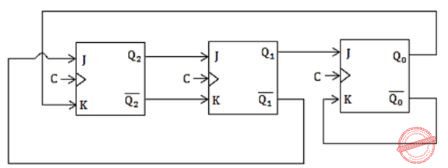The above synchronous sequential circuit built using JK flip-flops is initialized with Q2Q1Q0 = 000. The state sequence for this circuit for the next 3 clock cycles is

 A 001, 010, 011 B 111, 110, 101 C 100, 110, 111 D 100, 011, 001
Digital-Logic-Design       Flip-Flops
Question 55 Explanation:Question 56

With respect to the numerical evaluation of the definite integral,  where a  and b are given, which of the following statements is/are TRUE?

I) The value of obtained using the trapezoidal rule is always greater than or equal to the exact value of the definite integral.
II) The value of obtained using the Simpson’s rule is always equal to the exact value of the definite integral.

 A I only B II only C Both I and II D Neither I nor II
Engineering-Mathematics       Calculus
Question 56 Explanation:
Note: Numerical methods are out of syllabus for the GATE -CS.
 Question 57

The value of the integral given below isA -2π B π C -π D 2π
Engineering-Mathematics       Calculus
Question 57 Explanation:Question 58

Let S be a sample space and two mutually exclusive events A and B be such that A∪B = S. If P(∙) denotes the probability of the event, the maximum value of P(A)P(B) is __________.

 A 0.25 B 0.26 C 0.27 D 0.28
Engineering-Mathematics       Probability
Question 58 Explanation:
We know that
P(A∪B) = P(A) + P(B) + P(A∩B) = 1 →①
But, as A and B are mutually exclusive events
P(A∩B) = 0
∴ P(A∪B) = P(A) + P(B) = 1 →②
Arithmetic mean of two numbers ≥ Geometric mean of those two numbers
(P(A)+P(B))/2 ≥ √(P(A)∙P(B))
1/2 ≥ √(P(A)∙P(B)) (∵from ②)
Squaring on both sides
1/4 ≥ P(A)∙P(B)
P(A)∙P(B) ≤ 1/4
∴ Maximum value of P(A)P(B) = 1/4 = 0.25
 Question 59

Consider the set of all functions f: {0,1, … ,2014} → {0,1, … ,2014} such that f(f(i)) = i, for all 0 ≤ i ≤ 2014. Consider the following statements:

P. For each such function it must be the case that for every i, f(i) = i.
Q. For each such function it must be the case that for some i, f(i) = i.
R. Each such function must be onto.

Which one of the following is CORRECT?

 A P, Q and R are true B Only Q and R are true C Only P and Q are true D Only R is true
Engineering-Mathematics       Set-Theory
Question 59 Explanation:
f: {0,1,…,2014} → {0,1,…,2014} and f(f(i)) = iSo f(i)should be resulting only {0, 1, …2014}
So, every element in range has a result value to domain. This is onto. (Option R is correct)
We have ‘2015’ elements in domain.
So atleast one element can have f(i) = i,
so option ‘Q’ is also True.
∴ Q, R are correct.
 Question 60

There are two elements x, y in a group (G,∗) such that every element in the group can be written as a product of some number of x's and y's in some order. It is known that

`  x * x = y * y = x * y * x = y * x * y * x = e   `

where e is the identity element. The maximum number of elements in such a group is ________.

 A 4 B 5 C 6 D 7
Engineering-Mathematics       Graph-Theory
Question 60 Explanation:
We know
a*a-1 = e1. x*x = e So x-1 is x ⇒ x is element of Group
2. y*y = e So y-1 = y ⇒ y is element of Group4. (y*x)*(y*x) = x*y*y*x = x*x*e = e So (y*x)-1 = (y*x)
In ③, ④
x*y, y*x has same inverse, there should be unique inverse for each element.
x*y = y*x (even with cumulative law, we can conclude)
So {x, y, e, x*y} are element of Group.
 Question 61

If G is a forest with n vertices and k connected components, how many edges does G have?

 A ⌊n/k⌋ B ⌈n/k⌉ C n–k D n-k+1
Engineering-Mathematics       Graph-Theory
Question 61 Explanation:
Suppose, if each vertex is a component, then k=n, then there will not be any edges among them Replace the same in options
Option 1, 2 will give answer 1. (i.e. one edge among them),
Option 3: n-k = 0 edges.
Option 4: n-k+1 = 1 edge, which is false.
 Question 62

Let δ denote the minimum degree of a vertex in a graph. For all planar graphs on n vertices with δ ≥ 3, which one of the following is TRUE?

 A In any planar embedding, the number of faces is at least n/2 + 2 B In any planar embedding, the number of faces is less than n/2 + 2 C There is a planar embedding in which the number of faces is less than n/2 + 2 D There is a planar embedding in which the number of faces is at most n/(δ+1)
Engineering-Mathematics       Set-Theory
Question 62 Explanation:
Euler’s formula:
v – e + f = 2 →①
Point ① degree of each vertex is minimum ‘3’.3×n ≥ 2e
e ≤ 3n/2
From ① :
n-3n/2+f = 2 ⇒Question 63

The CORRECT formula for the sentence, “not all rainy days are cold” is

 A ∀d (Rainy(d) ∧∼Cold(d)) B ∀d (∼Rainy(d) → Cold(d)) C ∃d (∼Rainy(d) → Cold(d)) D ∃d (Rainy(d) ∧∼Cold(d))
Engineering-Mathematics       Prepositional-Logic
Question 63 Explanation:
Not all rainy days are cold
= ∼[∀rainy days are cold]
= ∼[∀ days (rainy days ⇒ cold days]
= ∃ days[∼(cold days ∨ ∼rainy days)]
= ∃ days[rainy days ∧ ∼cold days]
 Question 64

Consider the following relational schema:

```  employee(empId, empName, empDept)
customer(custId, custName, salesRepId, rating)```

salesRepId is a foreign key referring to empId of the employee relation. Assume that each employee makes a sale to at least one customer. What does the following query return?

```SELECT empName
FROM employee E
WHERE NOT EXISTS (SELECT custId
FROM customer C
WHERE C.salesRepId = E.empId
AND C.rating <> `GOOD`); ```
 A Names of all the employees with at least one of their customers having a ‘GOOD’ rating. B Names of all the employees with at most one of their customers having a ‘GOOD’ rating. C Names of all the employees with none of their customers having a ‘GOOD’ rating. D Names of all the employees with all their customers having a ‘GOOD’ rating.
Database-Management-System       SQL
Question 64 Explanation:The inner query i.e., ② represents all customers having other than ‘GOOD’ while the entire query represents name of all employees with all their customers having a ‘good rating’.
 Question 65

Let ⊕ denote the Exclusive OR (XOR) operation. Let ‘1’ and ‘0’ denote the binary constants. Consider the following Boolean expression for F over two variables P and Q:

`     F(P,Q) = ((1⊕P)⊕(P⊕Q))⊕((P⊕Q)⊕(Q⊕0)) `

The equivalent expression for F is

 A P+Q B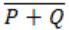C P⨁Q DDigital-Logic-Design       Number-Systems
Question 65 Explanation:
((1 ⊕ P) ⊕ (P ⊕ Q)) ⊕ ((P ⊕ Q) ⊕ (Q ⊕ 0))
⊕ is associative i.e P ⊕ (Q ⊕ R) = (P⊕Q) ⊕ R.
P ⊕ P = 0, 1 ⊕ P = P’ and 0 ⊕ Q = Q
(1 ⊕ P) ⊕ ((P ⊕ Q) ⊕ (P ⊕ Q)) ⊕ (Q ⊕ 0)
= P’⊕ (0) ⊕ Q
= P’ ⊕ Q
= (P ⊕ Q)’
There are 65 questions to complete.